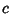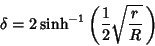## Inversive Distance

The inversive distance is the Natural Logarithm of the ratio of two concentric circles into which the given circles can be inverted. Letbe the distance between the centers of two nonintersecting Circles of Radiiand. Then the inversive distance is(Coxeter and Greitzer 1967).

The inversive distance between the Soddy Circles is given byand the Circumcircle and Incircle of a Triangle with Circumradiusand Inradiusare at inversive distance(Coxeter and Greitzer 1967, pp. 130-131).

References

Coxeter, H. S. M. and Greitzer, S. L. Geometry Revisited. Washington, DC: Math. Assoc. Amer., pp. 123-124 and 127-131, 1967.